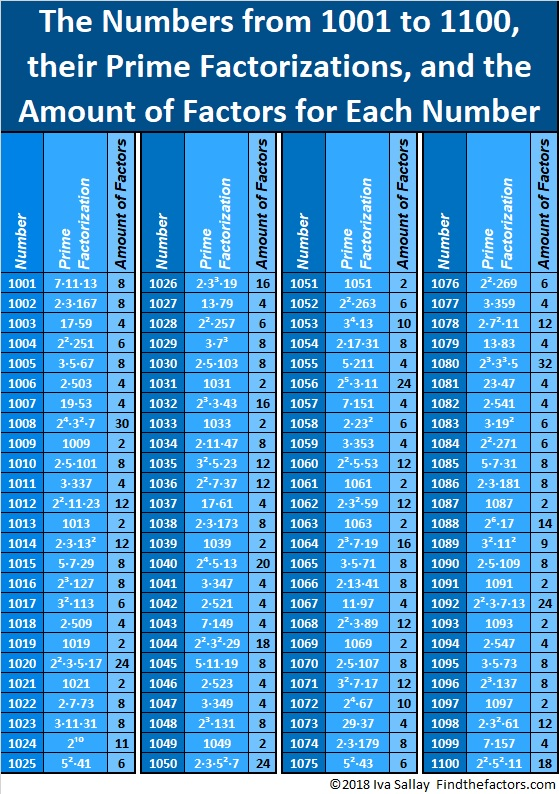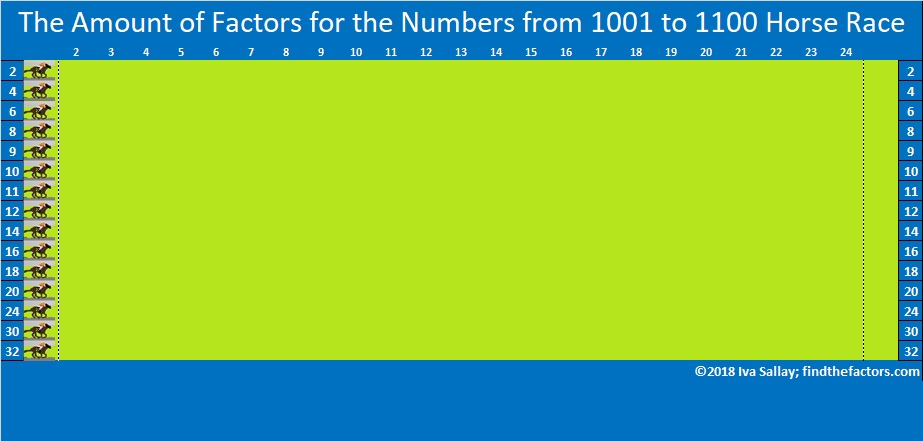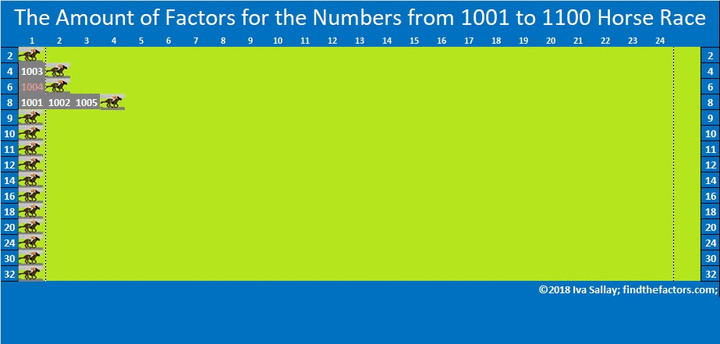# 1100 Now THIS Is a Horse Race!

Most people know that any prime number has exactly 2 factors. Every composite number has more than 2 factors. I’ve made a chart showing the numbers from 1001 to 1100, their prime factorizations, and the amount of factors each of those numbers has.The fewest factors any of those numbers have is 2 while the most factors any of the numbers have is 32. Which number of factors appears most often? Let’s have a horse race to find out!

Several horses representing different amounts of factors are lined up for the race. Spoiler alert: some of the horses will barely make it out of the gate. THIS will be an exciting horse race. There will be at least one lead change. You won’t know for sure which horse will cross the finish line first until the end. The second place horse will be SO close to winning.

I haven’t made a horse race this exciting since the 601 to 700 Horse Race, so pick your pony, then scroll down and see how your pony does!Click on the gif below to make the horse race larger.make science GIFs like this at MakeaGif

How did your pony do? Were you surprised by the results of the race?

Only perfect squares can have an odd number of factors which explains why 9 and 11 barely make it out of the gate. The numbers in pink have square factors so their square roots can be simplified. That describes 41 of the numbers from 1001 to 1100.

I hope this horse race sparks your curiosity about numbers! Each number is fascinating in its own way.

Let me tell you a few things specifically about the number 1100:

• 1100 is a composite number.
• Prime factorization: 1100 = 2 × 2 × 5 × 5 × 11, which can be written 1100 = 2² × 5² × 11
• The exponents in the prime factorization are 2, 2 and 1. Adding one to each and multiplying we get (2 + 1)(2 + 1)(1 + 1) = 3 × 3 × 2 = 18. Therefore 1100 has exactly 18 factors.
• Factors of 1100: 1, 2, 4, 5, 10, 11, 20, 22, 25, 44, 50, 55, 100, 110, 220, 275, 550, 1100
• Factor pairs: 1100 = 1 × 1100, 2 × 550, 4 × 275, 5 × 220, 10 × 110, 11 × 100, 20 × 55, 22 × 50 or 25 × 44
• Taking the factor pair with the largest square number factor, we get √1100 = (√100)(√11) = 10√11 ≈ 33.166251100 is the hypotenuse of two Pythagorean triples:
660-880-1100 which is (3-4-5) times 220
308-1056-1100 which is (7-24-25) times 44

It may interest you to know that 1100 is 3131 in BASE 7.
That’s because 3(7³) + 1(7²) + 3(7) + 1( 1) = 1100.

All those fun facts are straight from the horse’s mouth!

This site uses Akismet to reduce spam. Learn how your comment data is processed.